Question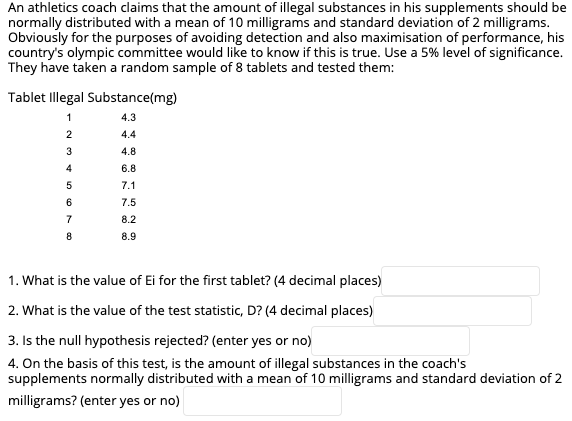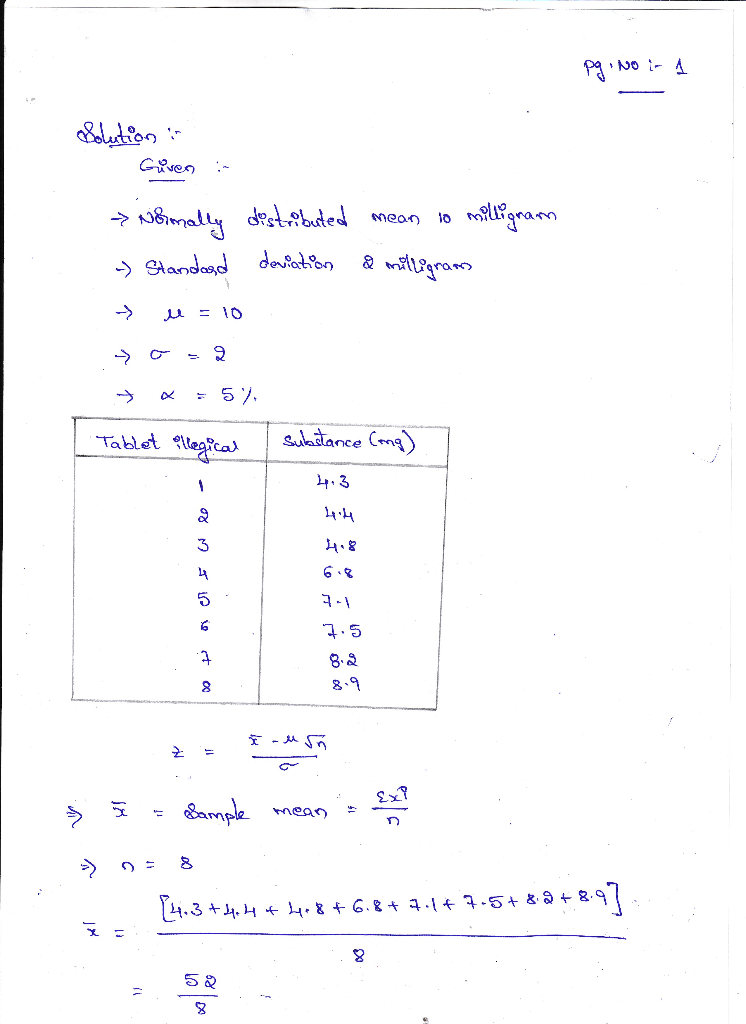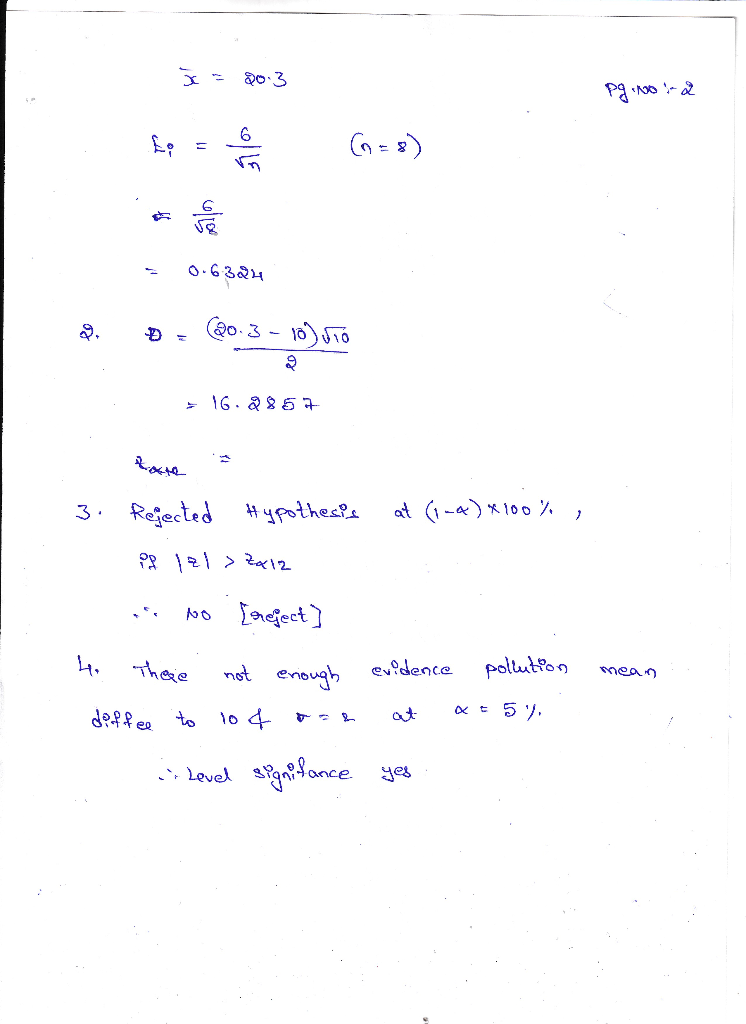#### Earn Coins

Coins can be redeemed for fabulous gifts.

Similar Homework Help Questions
• ### (1 point) Scores for men on the verbal portion of the SAT-I test are normally distributed...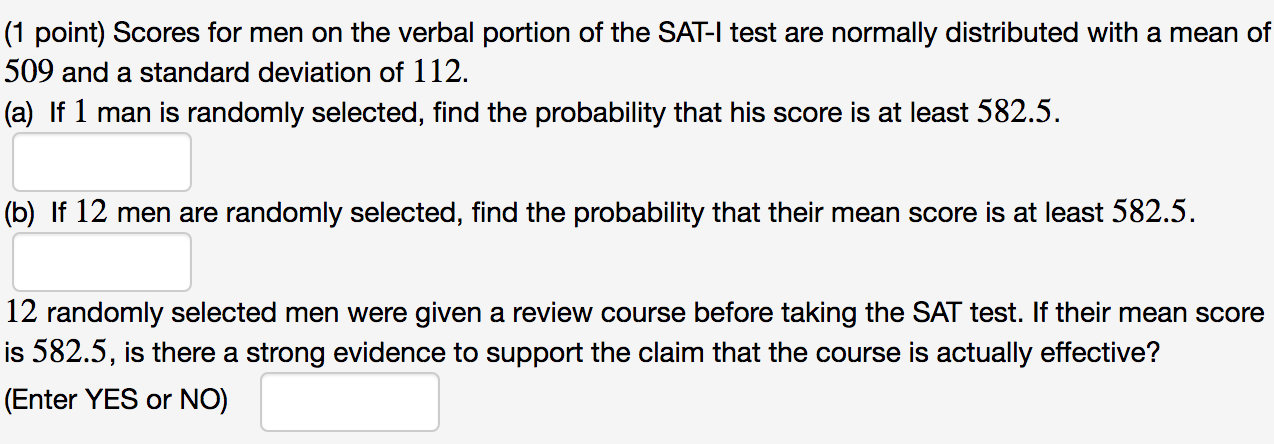(1 point) Scores for men on the verbal portion of the SAT-I test are normally distributed with a mean of 509 and a standard deviation of 112. (a) If 1 man is randomly selected, find the probability that his score is at least 582.5. (b) If 12 men are randomly selected, find the probability that their mean score is at least 582.5. 12 randomly selected men were given a review course before taking the SAT test. If their mean score...

• ### (1 point) Scores for men on the verbal portion of the SAT-I test are normally distributed...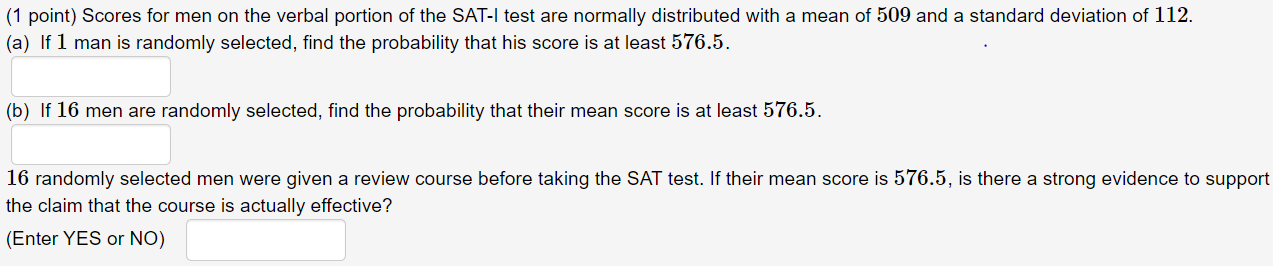(1 point) Scores for men on the verbal portion of the SAT-I test are normally distributed with a mean of 509 and a standard deviation of 112. (a) If 1 man is randomly selected, find the probability that his score is at least 576.5. (b) If 16 men are randomly selected, find the probability that their mean score is at least 576.5. 16 randomly selected men were given a review course before taking the SAT test. If their mean score...

• ### Scores for a common standardized college aptitude test are normally distributed with a mean of 480...

Scores for a common standardized college aptitude test are normally distributed with a mean of 480 and a standard deviation of 106. Randomly selected men are given a Test Preparation Course before taking this test. Assume, for sake of argument, that the preparation course has no effect. If 1 of the men is randomly selected, find the probability that his score is at least 553.4. P(X > 553.4) = Enter your answer as a number accurate to 4 decimal places....

• ### Scores for a common standardized college aptitude test are normally distributed with a mean of 515...

Scores for a common standardized college aptitude test are normally distributed with a mean of 515 and a standard deviation of 108. Randomly selected men are given a Test Preparation Course before taking this test. Assume, for sake of argument, that the preparation course has no effect. If 1 of the men is randomly selected, find the probability that his score is at least 554. P(X > 554) = Enter your answer as a number accurate to 4 decimal places....

• ### Scores for a common standardized college aptitude test are normally distributed with a mean of 491...

Scores for a common standardized college aptitude test are normally distributed with a mean of 491 and a standard deviation of 102. Randomly selected men are given a Test Preparation Course before taking this test. Assume, for sake of argument, that the preparation course has no effect. If 1 of the men is randomly selected, find the probability that his score is at least 555.6. P(X > 555.6) =   Enter your answer as a number accurate to 4 decimal places....

• ### Scores for a common standardized college aptitude test are normally distributed with a mean of 502...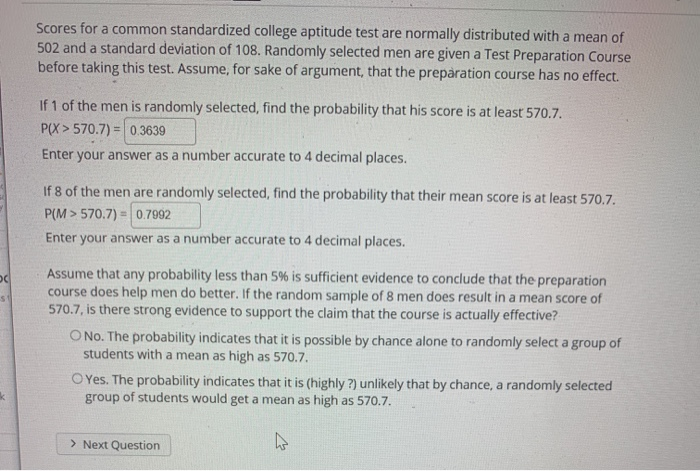Scores for a common standardized college aptitude test are normally distributed with a mean of 502 and a standard deviation of 108. Randomly selected men are given a Test Preparation Course before taking this test. Assume, for sake of argument that the preparation course has no effect. If 1 of the men is randomly selected, find the probability that his score is at least 570.7. P(X> 570.7) = 0.3639 Enter your answer as a number accurate to 4 decimal places....

• ### Scores for a common standardized college aptitude test are normally distributed with a mean of 484...

Scores for a common standardized college aptitude test are normally distributed with a mean of 484 and a standard deviation of 113. Randomly selected men are given a Test Preparation Course before taking this test. Assume, for sake of argument, that the preparation course has no effect. If 1 of the men is randomly selected, find the probability that his score is at least 582.2. A)P(X > 582.2) = (Enter your answer as a number accurate to 4 decimal places.)...

• ### Look at image, thank you. Scores for a common standardized college aptitude test are normally distributed...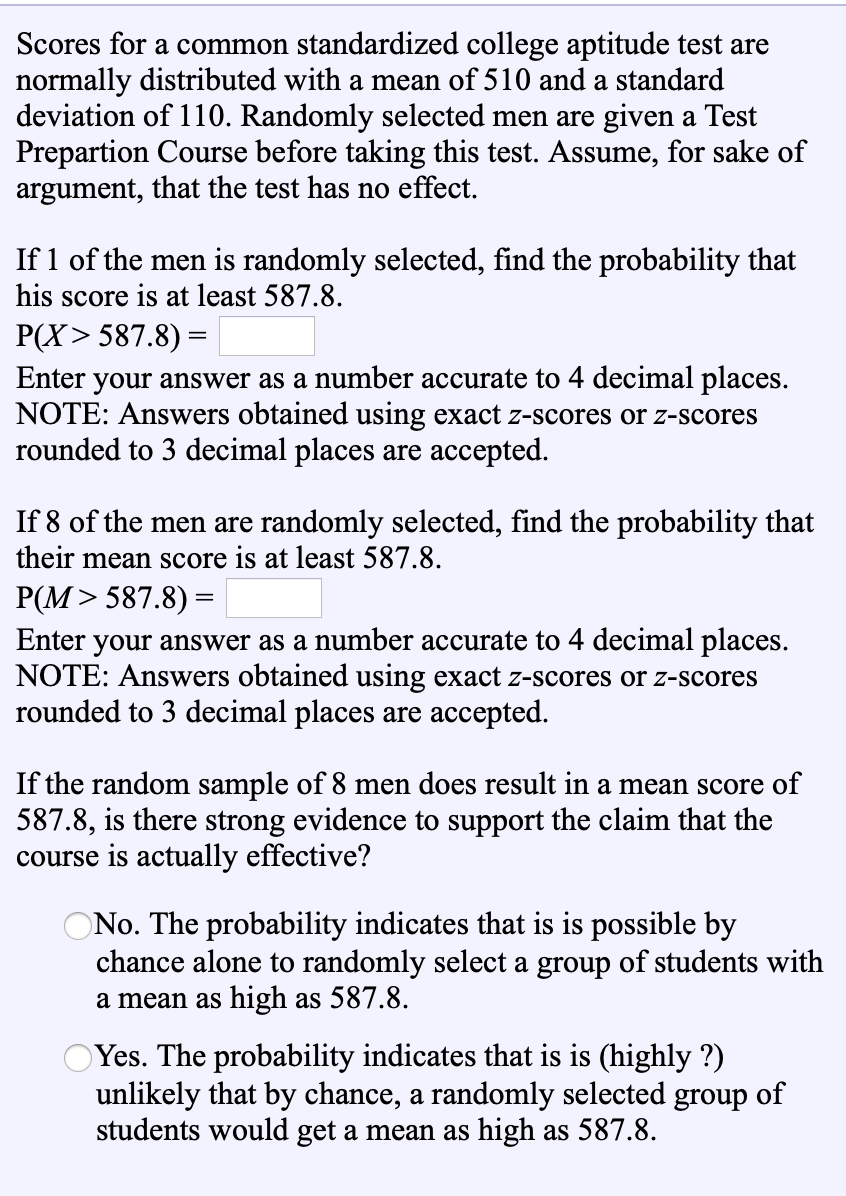Look at image, thank you. Scores for a common standardized college aptitude test are normally distributed with a mean of 510 and a standard deviation of 110. Randomly selected men are given a Test Prepartion Course before taking this test. Assume, for sake of argument, that the test has no effect. If 1 of the men is randomly selected, find the probability that his score is at least 587.8. P(X> 587.8) = Enter your answer as a number accurate to...